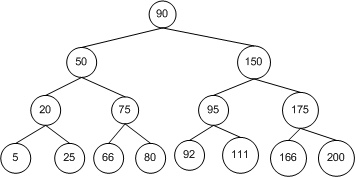## 2015年10月31日 星期六

### [Python] Are the two binary trees equal?Previous blog introduced how to implement a binary tree in Python[Python] Inplement Binary Tree，Today I’ll introduce how to determine if two binary trees are equal.
If two binary trees are equal, they are structurally identical and the nodes have the same value. Considering the definition above, we use dynamic function to test the two trees if they are equal.

``````# Definition for a binary tree node.
# class TreeNode(object):
#     def __init__(self, x):
#         self.val = x
#         self.left = None
#         self.right = None

class Solution(object):
def isSameTree(self, p, q):
"""
:type p: TreeNode
:type q: TreeNode
:rtype: bool
"""
##They are the same if they are None.
if not p and not q:
return True

if not p or not q:
return False

#For rest situations, the function will test if two input nodes have the same value, and use the function itself to the right and left nodes. It will return False if any conditions is return Fasle.

return p.val == q.val and self.isSameTree(p.right, q.right) and self.isSameTree(p.left,q.left)``````
﻿